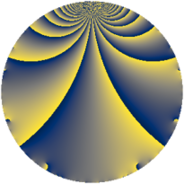# Properties

 Label 1911.1.h.aLevel $1911$ Weight $1$ Character orbit 1911.h Self dual yes Analytic conductor $0.954$ Analytic rank $0$ Dimension $1$ Projective image $D_{2}$ CM/RM discs -3, -39, 13 Inner twists $4$

# Related objects

## Newspace parameters

 Level: $$N$$ $$=$$ $$1911 = 3 \cdot 7^{2} \cdot 13$$ Weight: $$k$$ $$=$$ $$1$$ Character orbit: $$[\chi]$$ $$=$$ 1911.h (of order $$2$$, degree $$1$$, minimal)

## Newform invariants

 Self dual: yes Analytic conductor: $$0.953713239142$$ Analytic rank: $$0$$ Dimension: $$1$$ Coefficient field: $$\mathbb{Q}$$ Coefficient ring: $$\mathbb{Z}$$ Coefficient ring index: $$1$$ Twist minimal: no (minimal twist has level 39) Projective image: $$D_{2}$$ Projective field: Galois closure of $$\Q(\sqrt{-3}, \sqrt{13})$$ Artin image: $D_4$ Artin field: Galois closure of 4.0.5733.1

## $q$-expansion

 $$f(q)$$ $$=$$ $$q + q^{3} - q^{4} + q^{9} + O(q^{10})$$ $$q + q^{3} - q^{4} + q^{9} - q^{12} + q^{13} + q^{16} - q^{25} + q^{27} - q^{36} + q^{39} + 2 q^{43} + q^{48} - q^{52} + 2 q^{61} - q^{64} - q^{75} - 2 q^{79} + q^{81} + O(q^{100})$$

## Character values

We give the values of $$\chi$$ on generators for $$\left(\mathbb{Z}/1911\mathbb{Z}\right)^\times$$.

 $$n$$ $$638$$ $$1471$$ $$1522$$ $$\chi(n)$$ $$-1$$ $$-1$$ $$1$$

## Embeddings

For each embedding $$\iota_m$$ of the coefficient field, the values $$\iota_m(a_n)$$ are shown below.

For more information on an embedded modular form you can click on its label.

Label $$\iota_m(\nu)$$ $$a_{2}$$ $$a_{3}$$ $$a_{4}$$ $$a_{5}$$ $$a_{6}$$ $$a_{7}$$ $$a_{8}$$ $$a_{9}$$ $$a_{10}$$
1520.1
 0
0 1.00000 −1.00000 0 0 0 0 1.00000 0
 $$n$$: e.g. 2-40 or 990-1000 Significant digits: Format: Complex embeddings Normalized embeddings Satake parameters Satake angles

## Inner twists

Char Parity Ord Mult Type
1.a even 1 1 trivial
3.b odd 2 1 CM by $$\Q(\sqrt{-3})$$
13.b even 2 1 RM by $$\Q(\sqrt{13})$$
39.d odd 2 1 CM by $$\Q(\sqrt{-39})$$

## Twists

By twisting character orbit
Char Parity Ord Mult Type Twist Min Dim
1.a even 1 1 trivial 1911.1.h.a 1
3.b odd 2 1 CM 1911.1.h.a 1
7.b odd 2 1 39.1.d.a 1
7.c even 3 2 1911.1.w.a 2
7.d odd 6 2 1911.1.w.b 2
13.b even 2 1 RM 1911.1.h.a 1
21.c even 2 1 39.1.d.a 1
21.g even 6 2 1911.1.w.b 2
21.h odd 6 2 1911.1.w.a 2
28.d even 2 1 624.1.l.a 1
35.c odd 2 1 975.1.g.a 1
35.f even 4 2 975.1.e.a 2
39.d odd 2 1 CM 1911.1.h.a 1
56.e even 2 1 2496.1.l.a 1
56.h odd 2 1 2496.1.l.b 1
63.l odd 6 2 1053.1.n.b 2
63.o even 6 2 1053.1.n.b 2
84.h odd 2 1 624.1.l.a 1
91.b odd 2 1 39.1.d.a 1
91.i even 4 2 507.1.c.a 1
91.n odd 6 2 507.1.h.a 2
91.r even 6 2 1911.1.w.a 2
91.s odd 6 2 1911.1.w.b 2
91.t odd 6 2 507.1.h.a 2
91.bc even 12 4 507.1.i.a 2
105.g even 2 1 975.1.g.a 1
105.k odd 4 2 975.1.e.a 2
168.e odd 2 1 2496.1.l.a 1
168.i even 2 1 2496.1.l.b 1
273.g even 2 1 39.1.d.a 1
273.o odd 4 2 507.1.c.a 1
273.u even 6 2 507.1.h.a 2
273.w odd 6 2 1911.1.w.a 2
273.ba even 6 2 1911.1.w.b 2
273.bn even 6 2 507.1.h.a 2
273.ca odd 12 4 507.1.i.a 2
364.h even 2 1 624.1.l.a 1
455.h odd 2 1 975.1.g.a 1
455.s even 4 2 975.1.e.a 2
728.b even 2 1 2496.1.l.a 1
728.l odd 2 1 2496.1.l.b 1
819.ce even 6 2 1053.1.n.b 2
819.cy odd 6 2 1053.1.n.b 2
1092.d odd 2 1 624.1.l.a 1
1365.g even 2 1 975.1.g.a 1
1365.bl odd 4 2 975.1.e.a 2
2184.y even 2 1 2496.1.l.b 1
2184.bf odd 2 1 2496.1.l.a 1

By twisted newform orbit
Twist Min Dim Char Parity Ord Mult Type
39.1.d.a 1 7.b odd 2 1
39.1.d.a 1 21.c even 2 1
39.1.d.a 1 91.b odd 2 1
39.1.d.a 1 273.g even 2 1
507.1.c.a 1 91.i even 4 2
507.1.c.a 1 273.o odd 4 2
507.1.h.a 2 91.n odd 6 2
507.1.h.a 2 91.t odd 6 2
507.1.h.a 2 273.u even 6 2
507.1.h.a 2 273.bn even 6 2
507.1.i.a 2 91.bc even 12 4
507.1.i.a 2 273.ca odd 12 4
624.1.l.a 1 28.d even 2 1
624.1.l.a 1 84.h odd 2 1
624.1.l.a 1 364.h even 2 1
624.1.l.a 1 1092.d odd 2 1
975.1.e.a 2 35.f even 4 2
975.1.e.a 2 105.k odd 4 2
975.1.e.a 2 455.s even 4 2
975.1.e.a 2 1365.bl odd 4 2
975.1.g.a 1 35.c odd 2 1
975.1.g.a 1 105.g even 2 1
975.1.g.a 1 455.h odd 2 1
975.1.g.a 1 1365.g even 2 1
1053.1.n.b 2 63.l odd 6 2
1053.1.n.b 2 63.o even 6 2
1053.1.n.b 2 819.ce even 6 2
1053.1.n.b 2 819.cy odd 6 2
1911.1.h.a 1 1.a even 1 1 trivial
1911.1.h.a 1 3.b odd 2 1 CM
1911.1.h.a 1 13.b even 2 1 RM
1911.1.h.a 1 39.d odd 2 1 CM
1911.1.w.a 2 7.c even 3 2
1911.1.w.a 2 21.h odd 6 2
1911.1.w.a 2 91.r even 6 2
1911.1.w.a 2 273.w odd 6 2
1911.1.w.b 2 7.d odd 6 2
1911.1.w.b 2 21.g even 6 2
1911.1.w.b 2 91.s odd 6 2
1911.1.w.b 2 273.ba even 6 2
2496.1.l.a 1 56.e even 2 1
2496.1.l.a 1 168.e odd 2 1
2496.1.l.a 1 728.b even 2 1
2496.1.l.a 1 2184.bf odd 2 1
2496.1.l.b 1 56.h odd 2 1
2496.1.l.b 1 168.i even 2 1
2496.1.l.b 1 728.l odd 2 1
2496.1.l.b 1 2184.y even 2 1

## Hecke kernels

This newform subspace can be constructed as the intersection of the kernels of the following linear operators acting on $$S_{1}^{\mathrm{new}}(1911, [\chi])$$:

 $$T_{2}$$ $$T_{61} - 2$$ $$T_{199} - 2$$

## Hecke characteristic polynomials

$p$ $F_p(T)$
$2$ $$T$$
$3$ $$-1 + T$$
$5$ $$T$$
$7$ $$T$$
$11$ $$T$$
$13$ $$-1 + T$$
$17$ $$T$$
$19$ $$T$$
$23$ $$T$$
$29$ $$T$$
$31$ $$T$$
$37$ $$T$$
$41$ $$T$$
$43$ $$-2 + T$$
$47$ $$T$$
$53$ $$T$$
$59$ $$T$$
$61$ $$-2 + T$$
$67$ $$T$$
$71$ $$T$$
$73$ $$T$$
$79$ $$2 + T$$
$83$ $$T$$
$89$ $$T$$
$97$ $$T$$